Successfully reported this slideshow.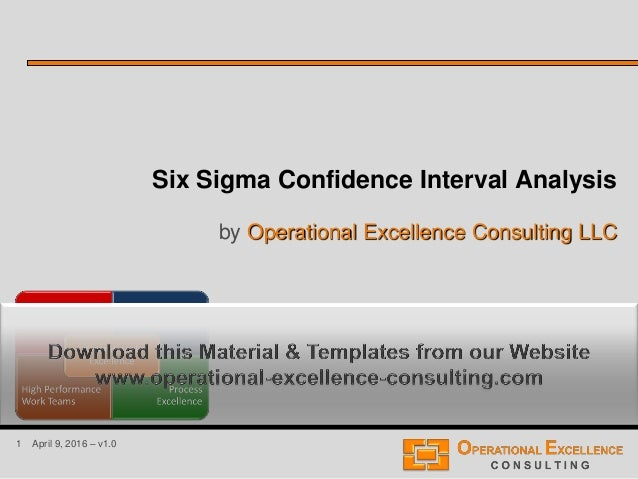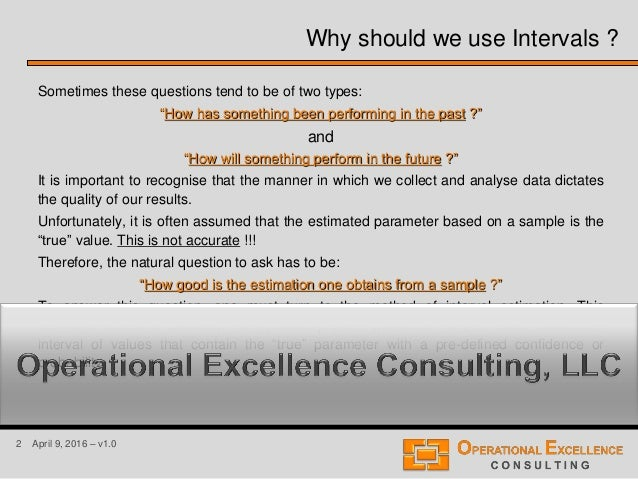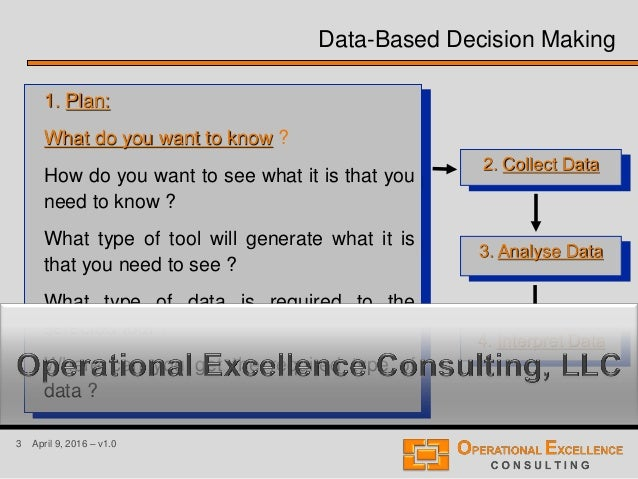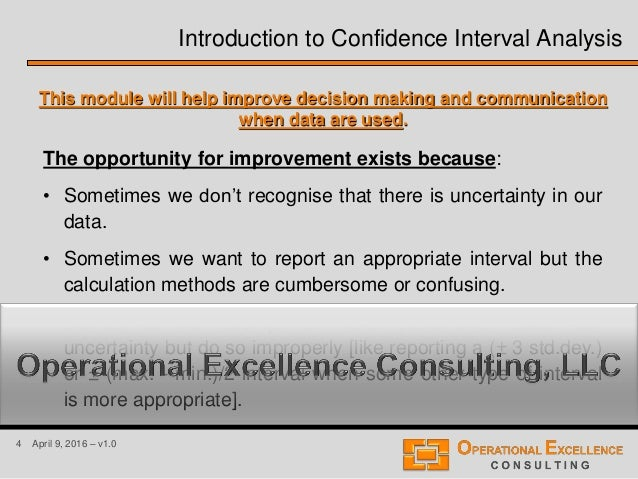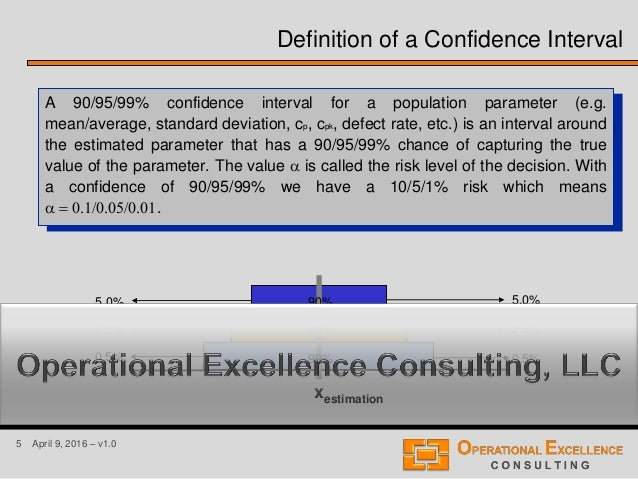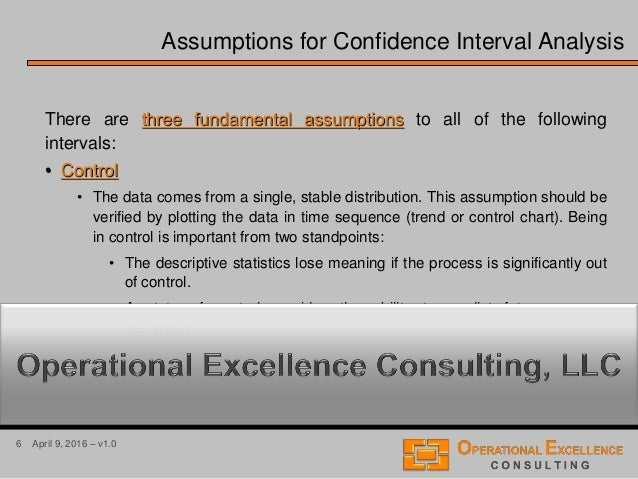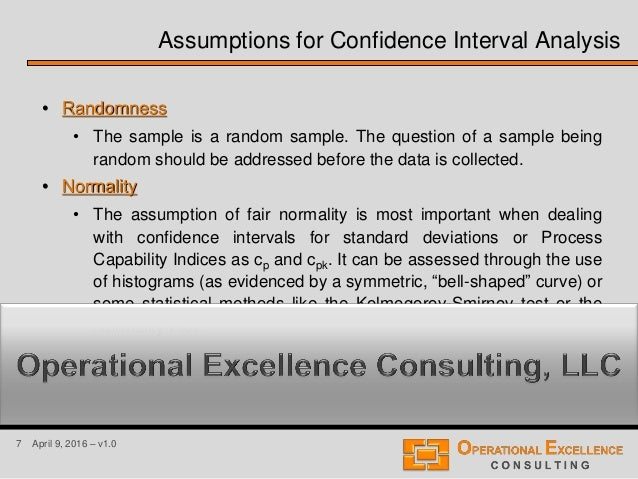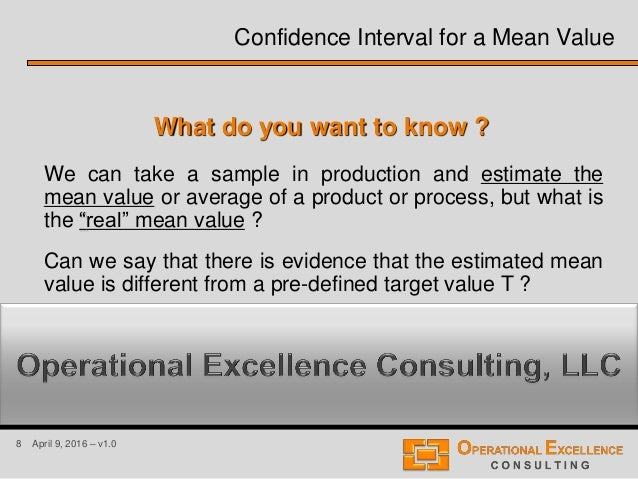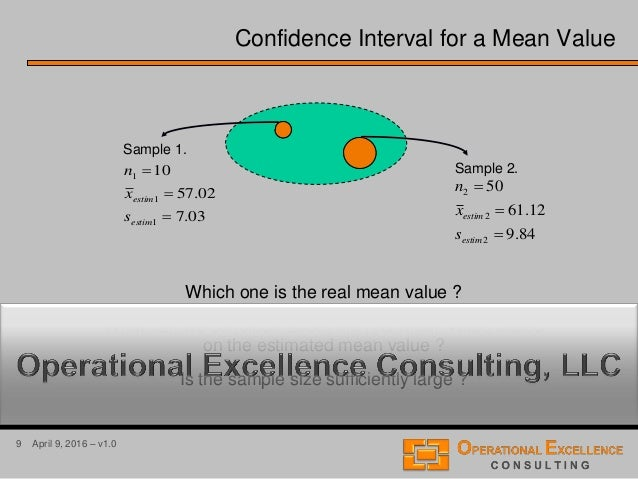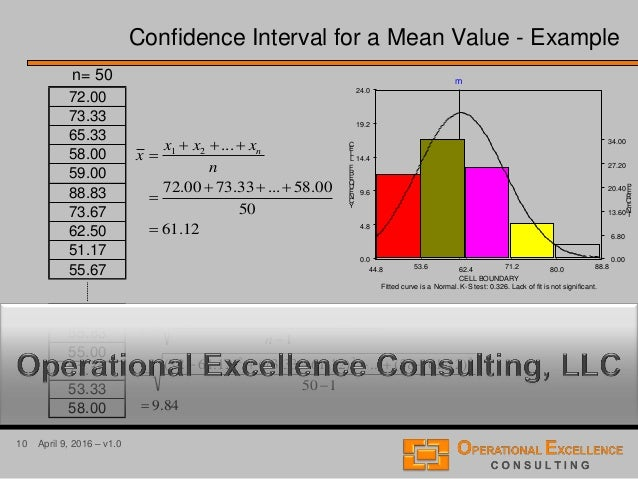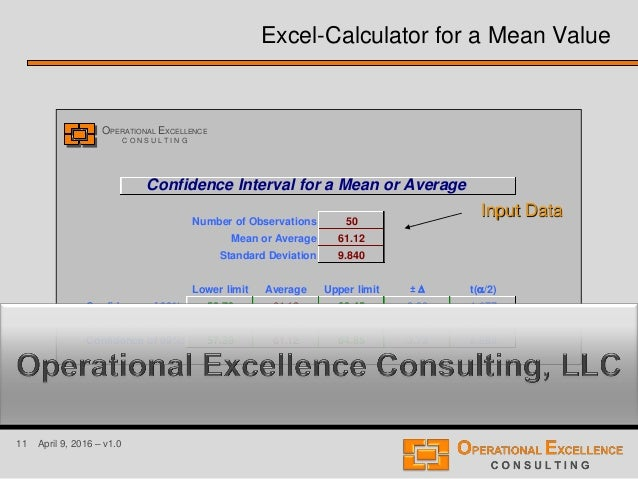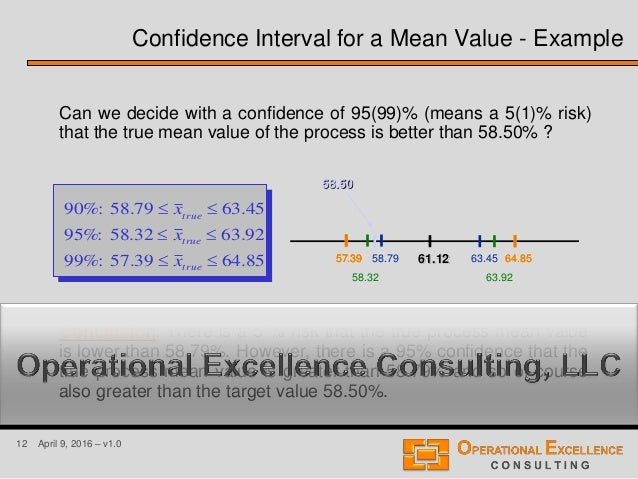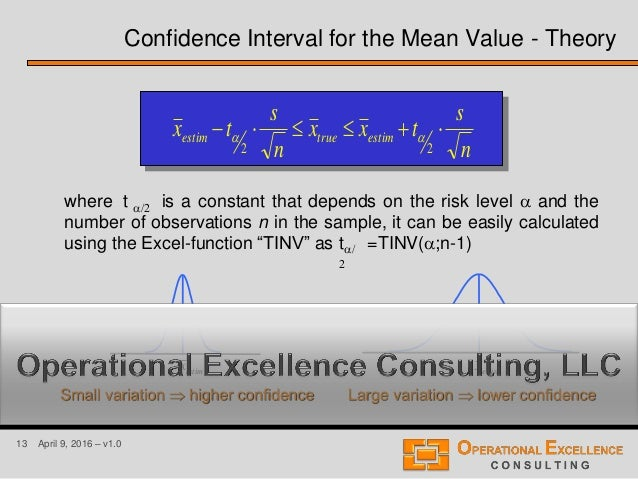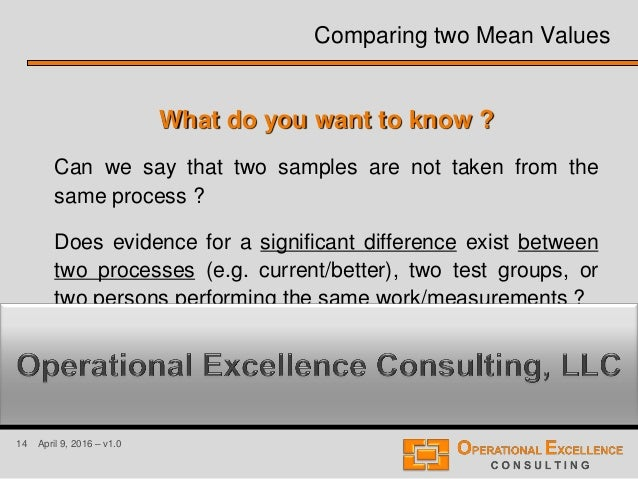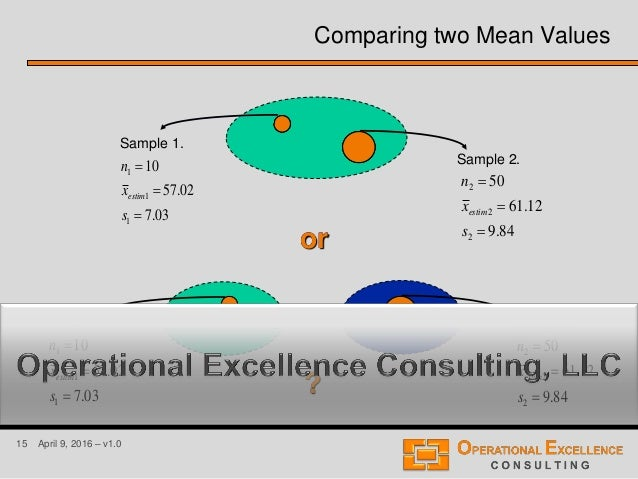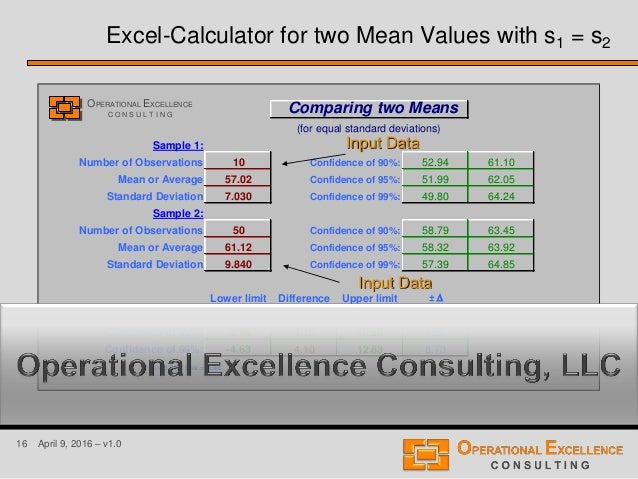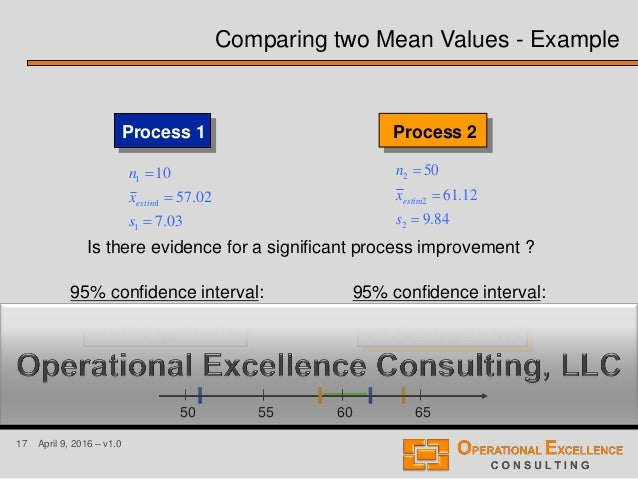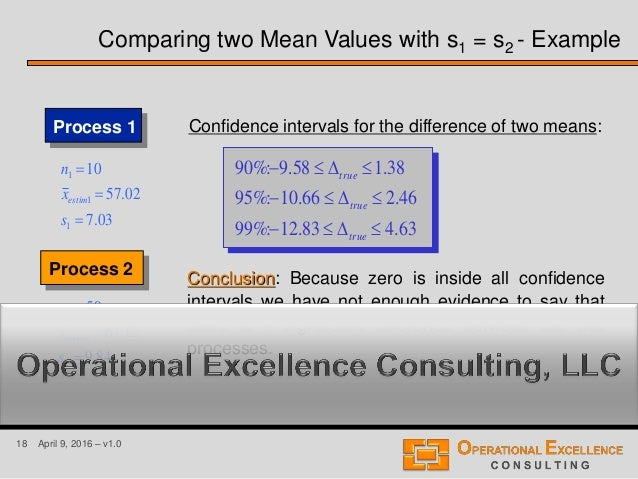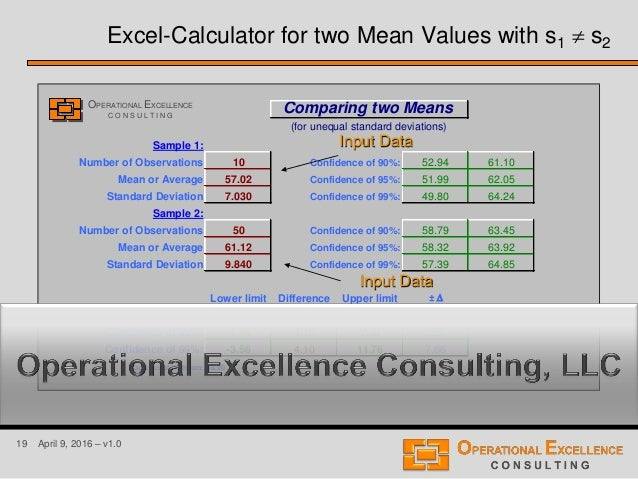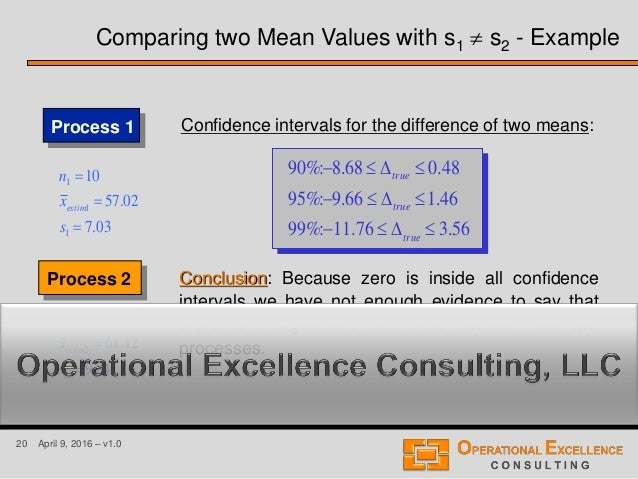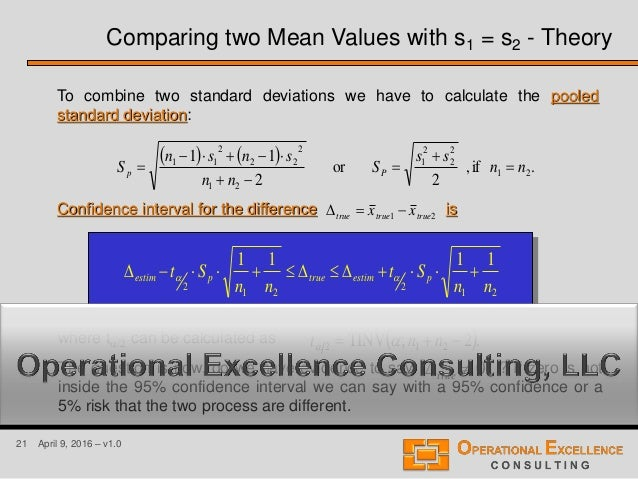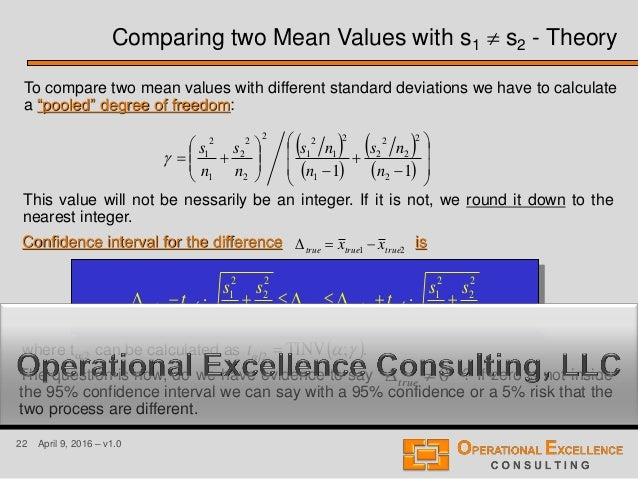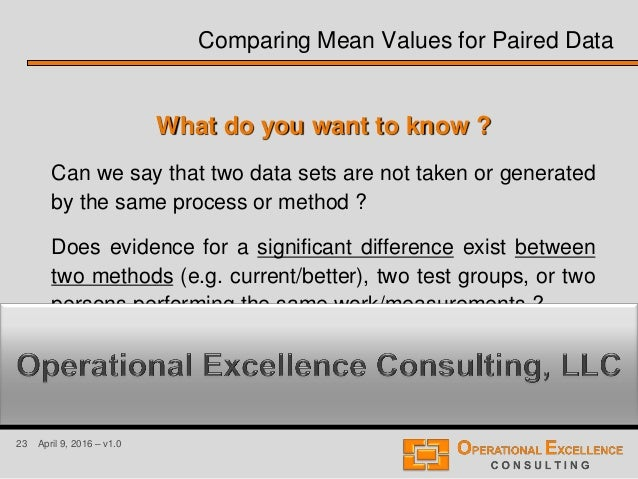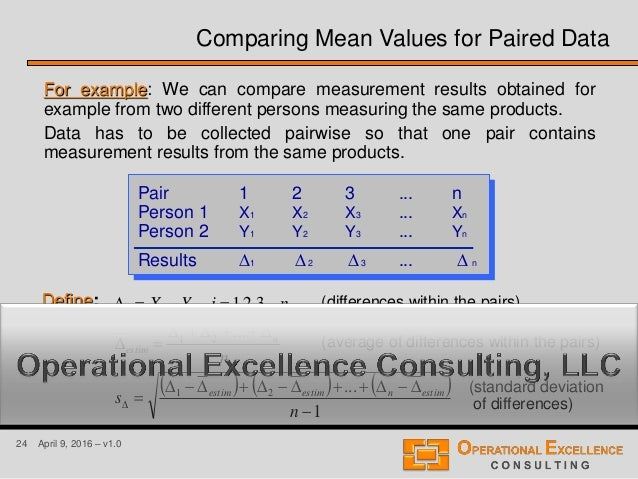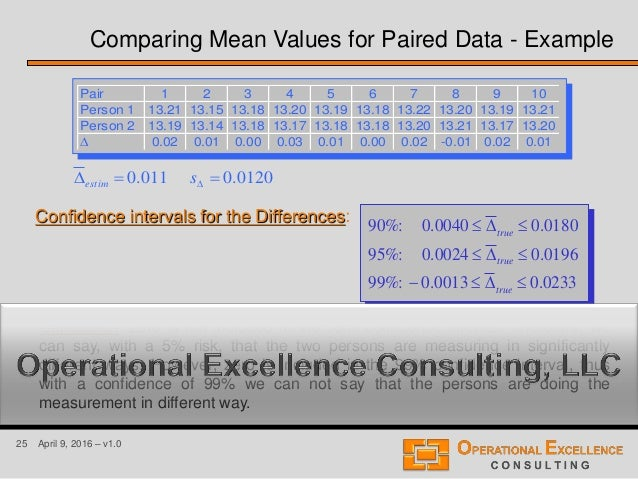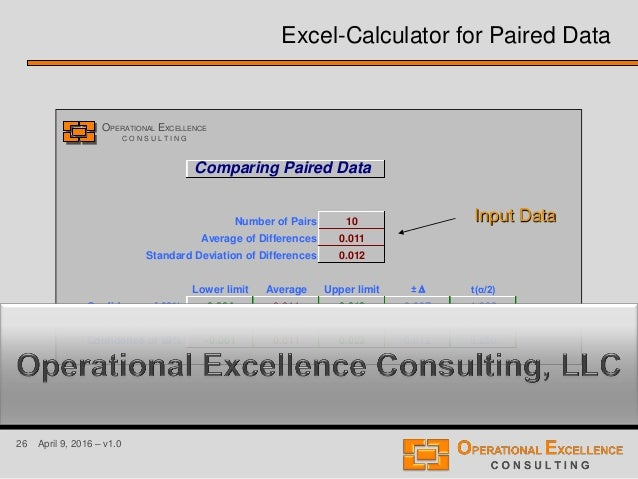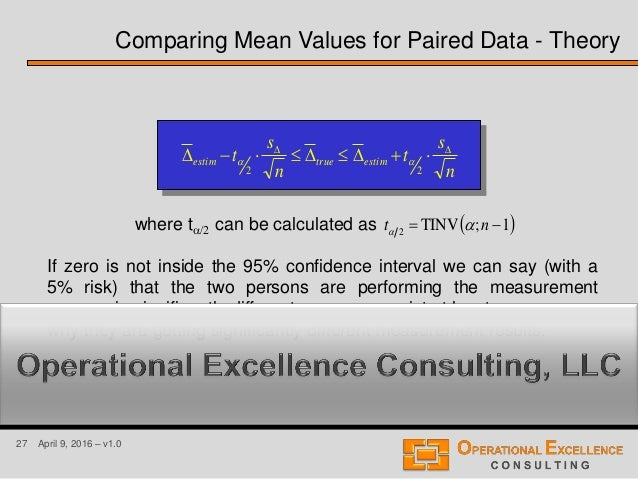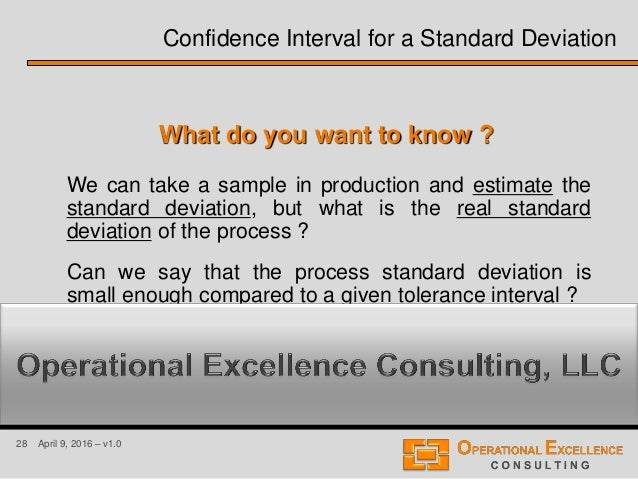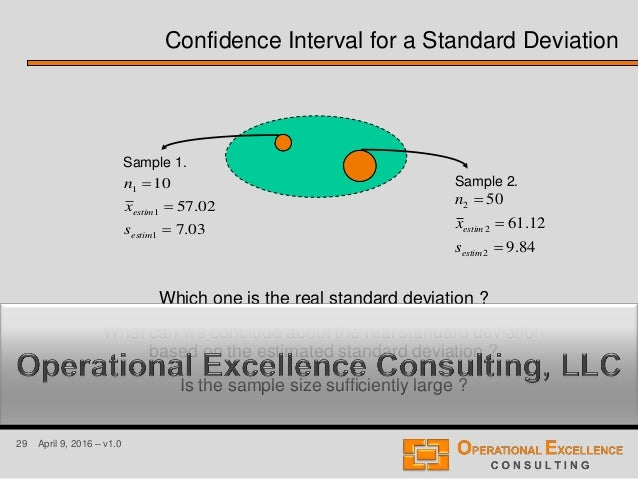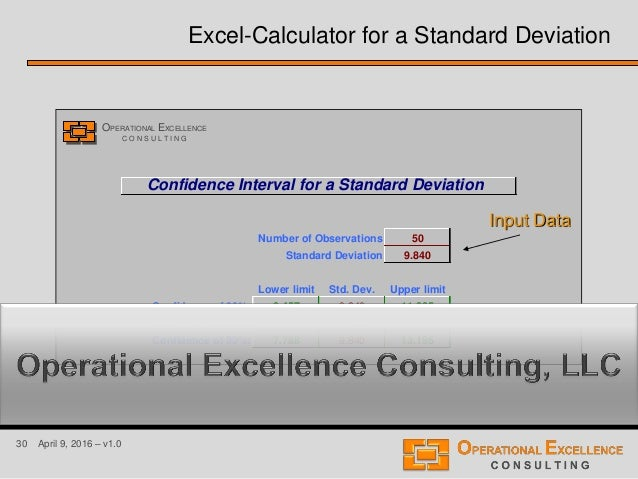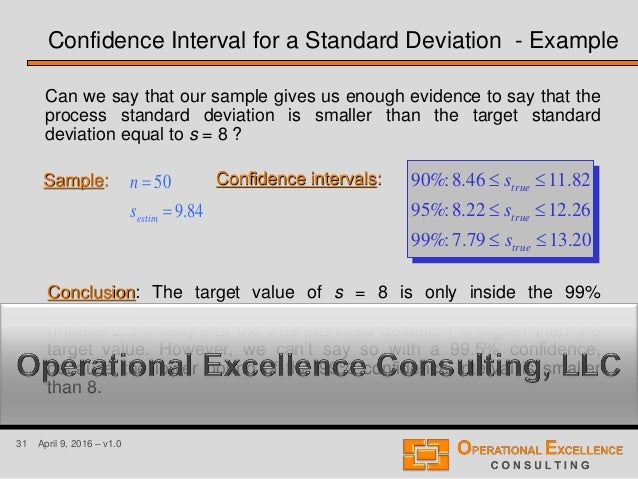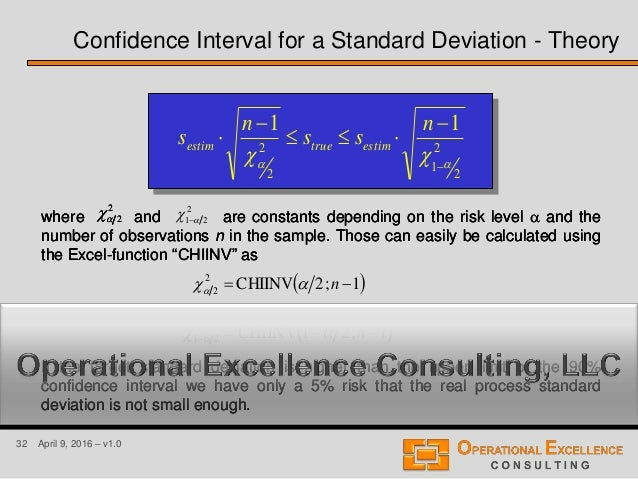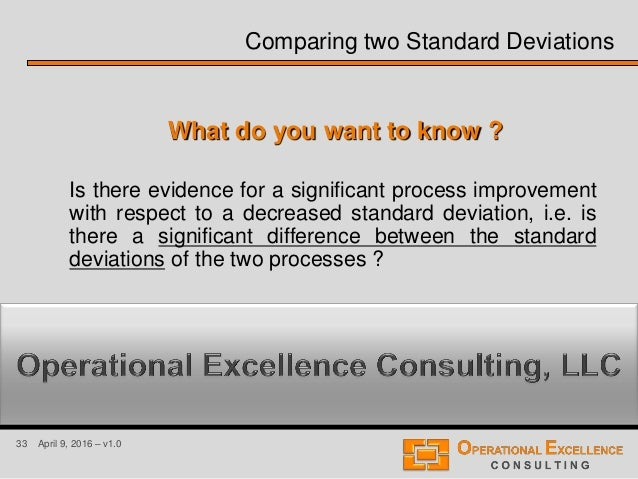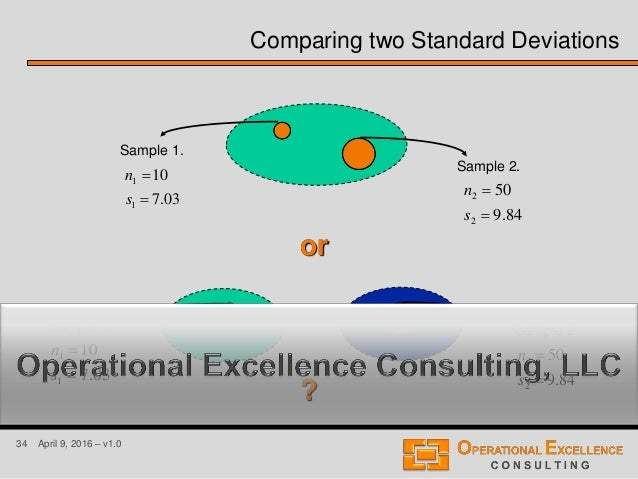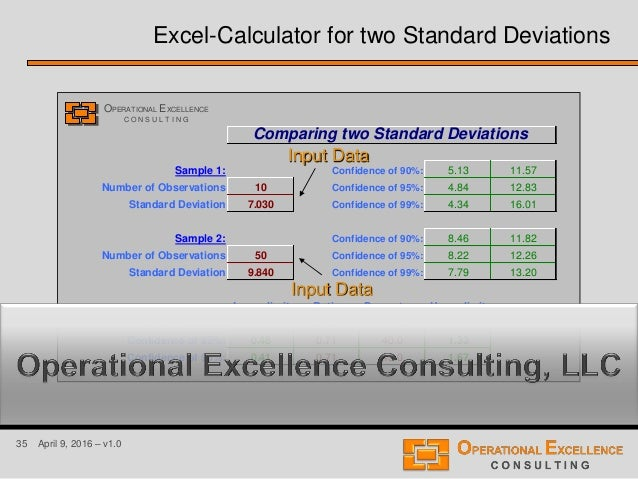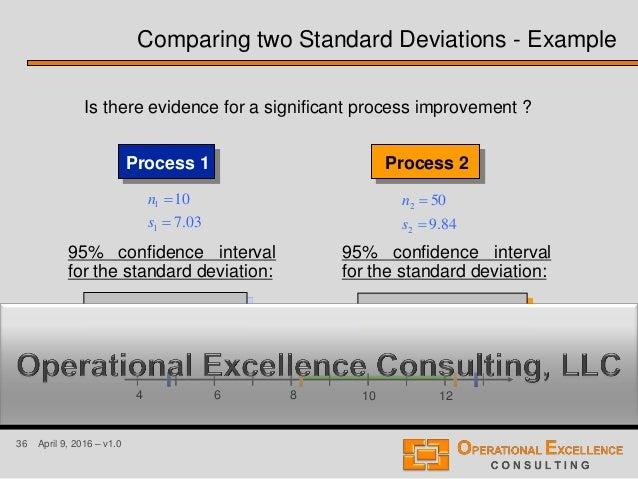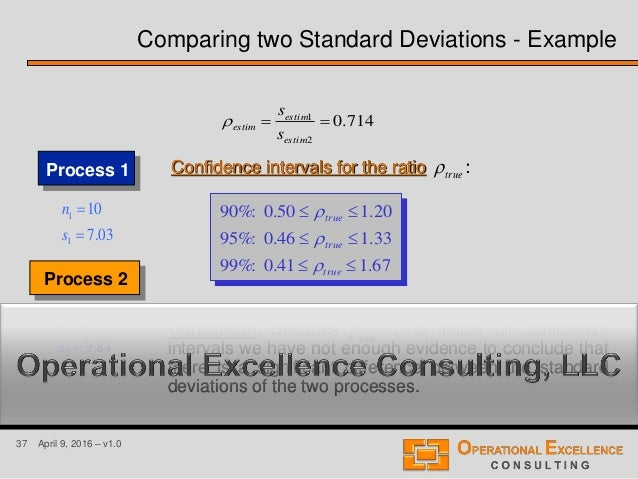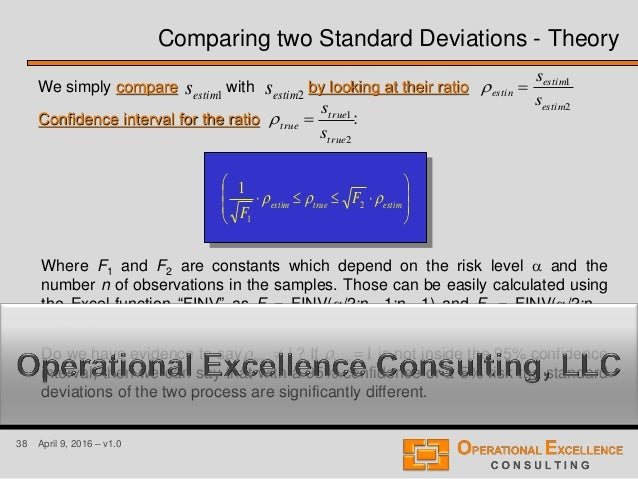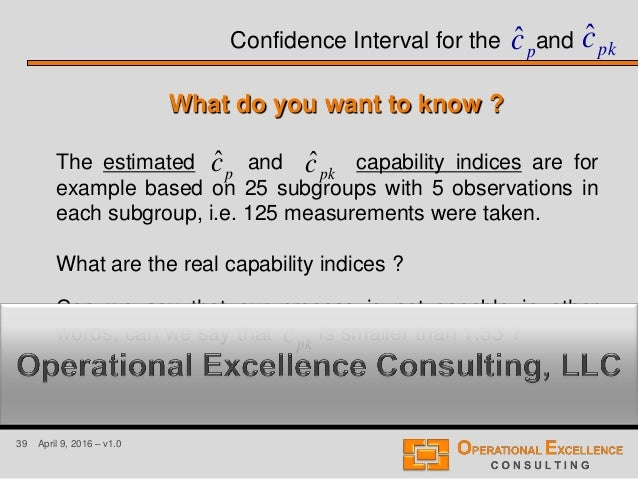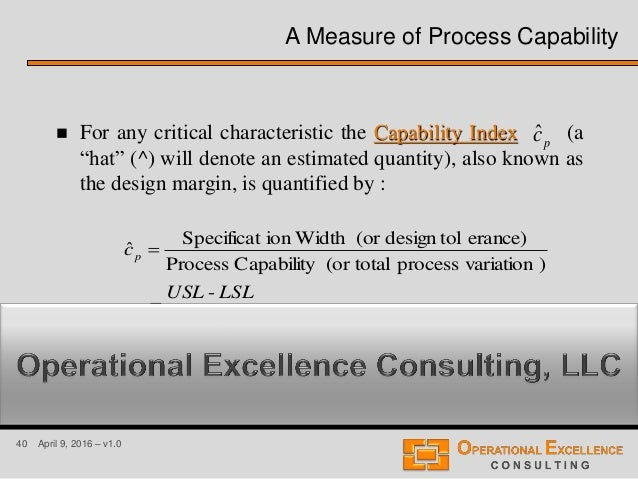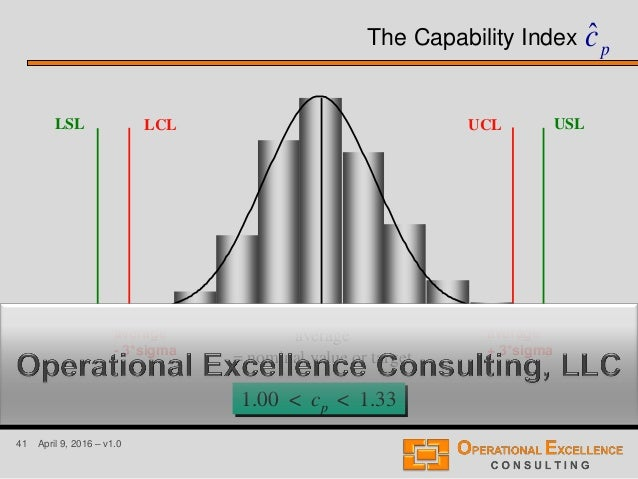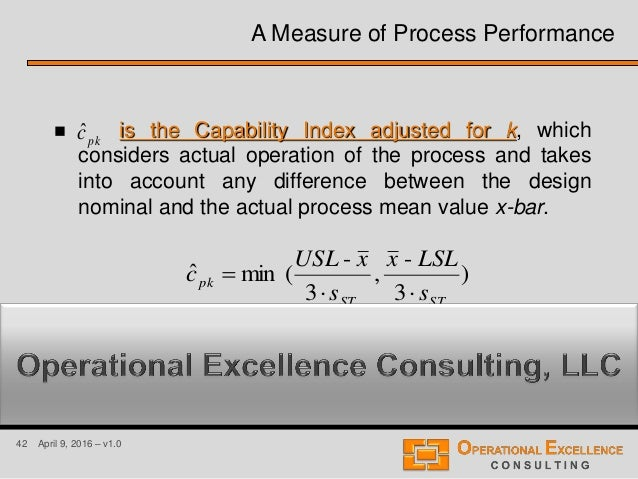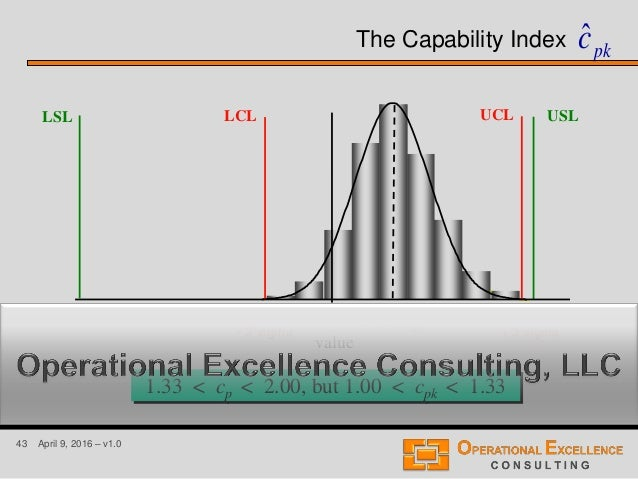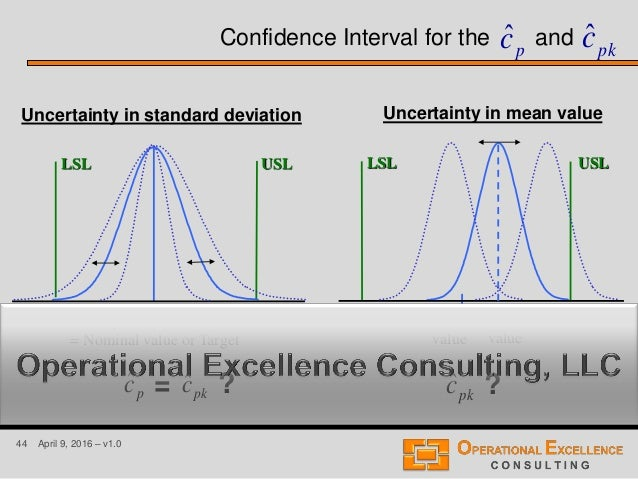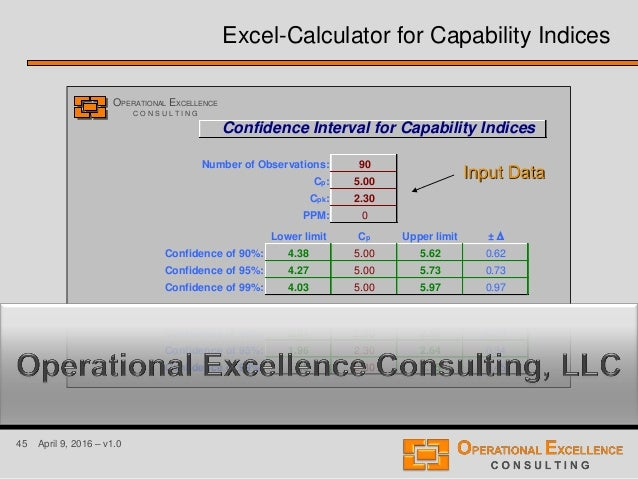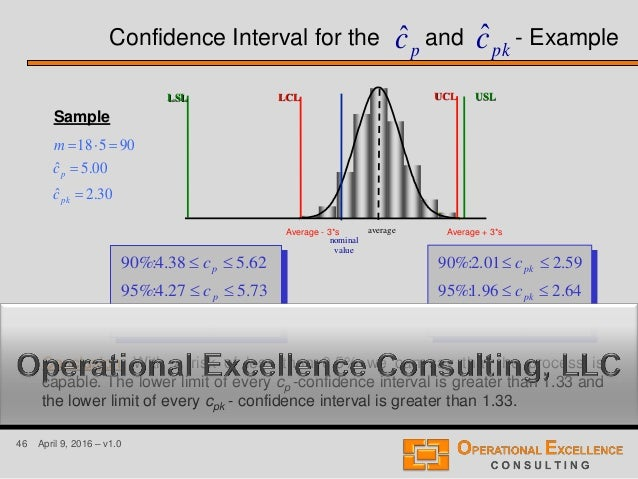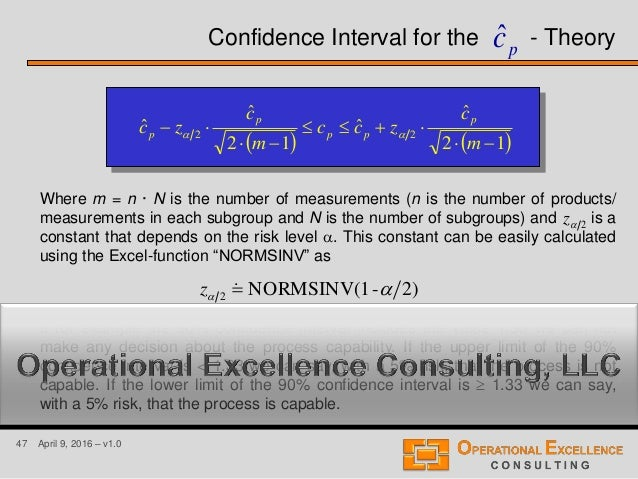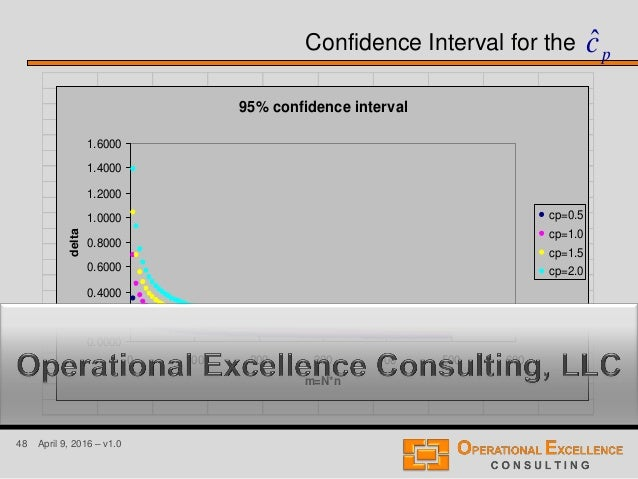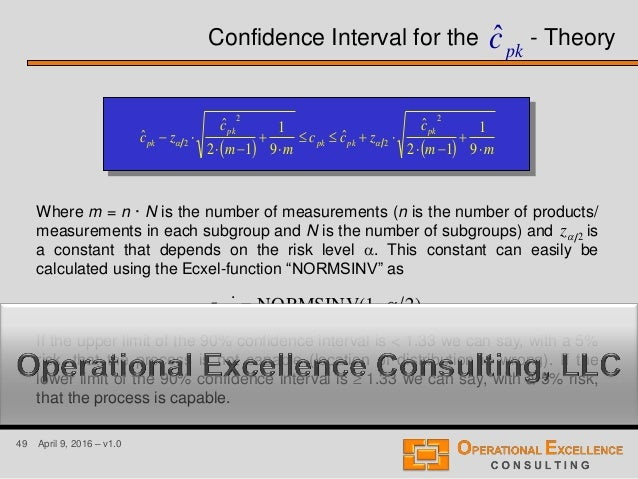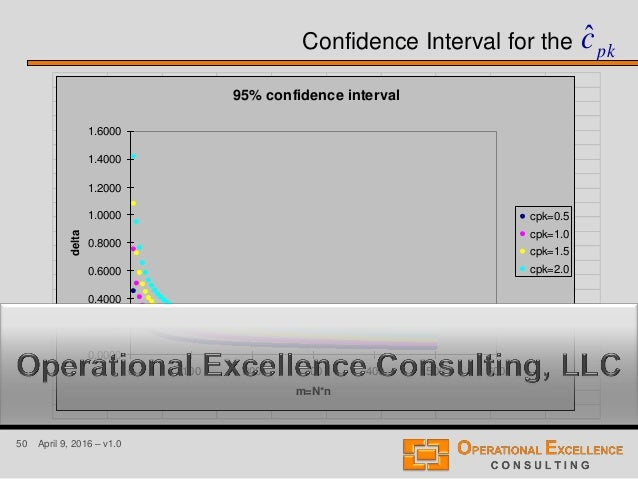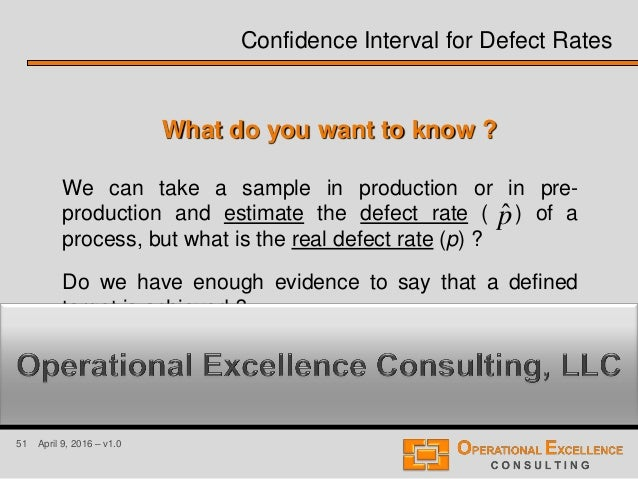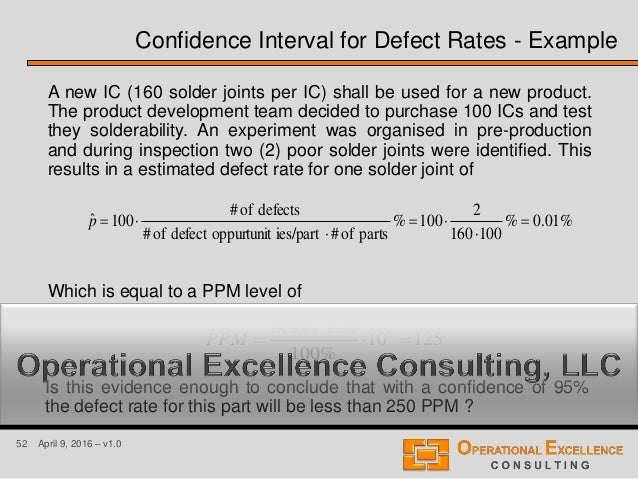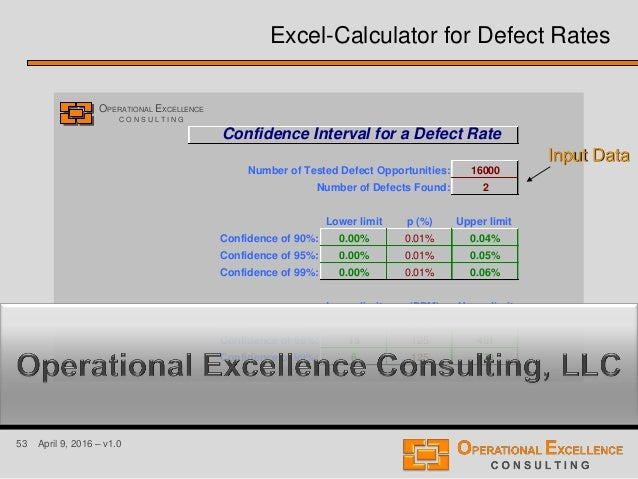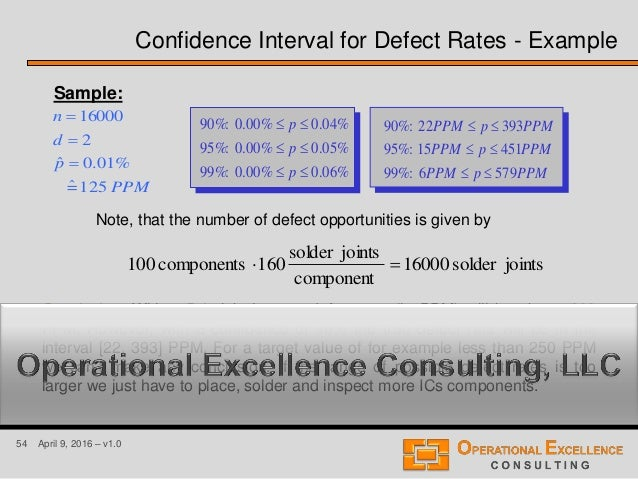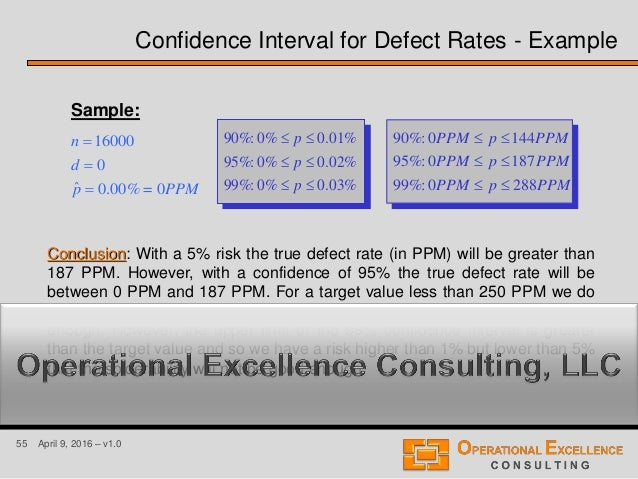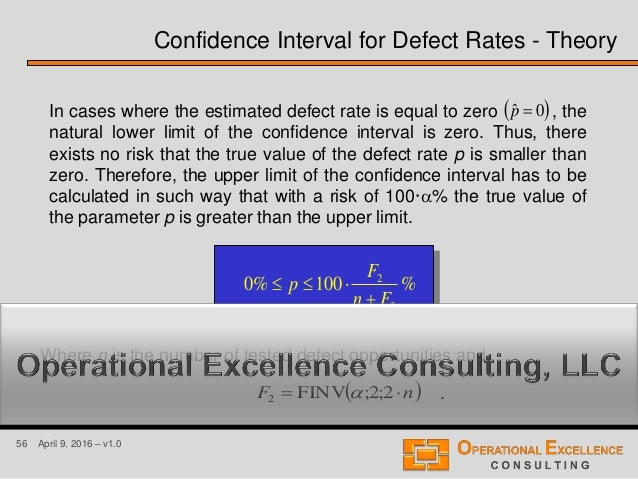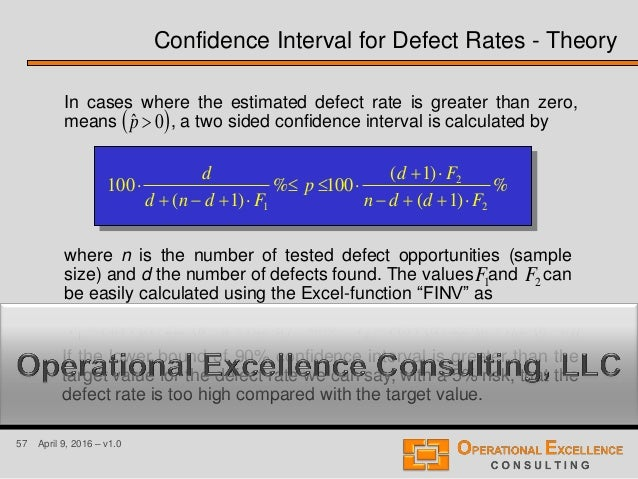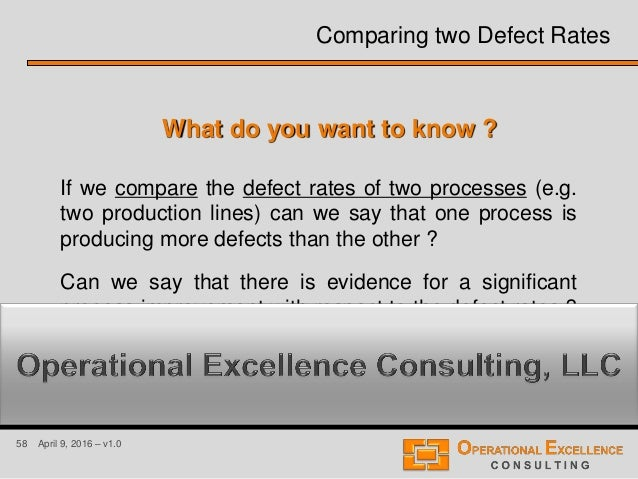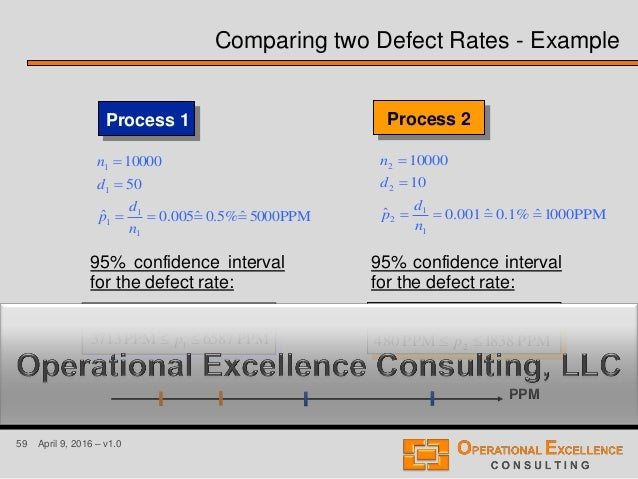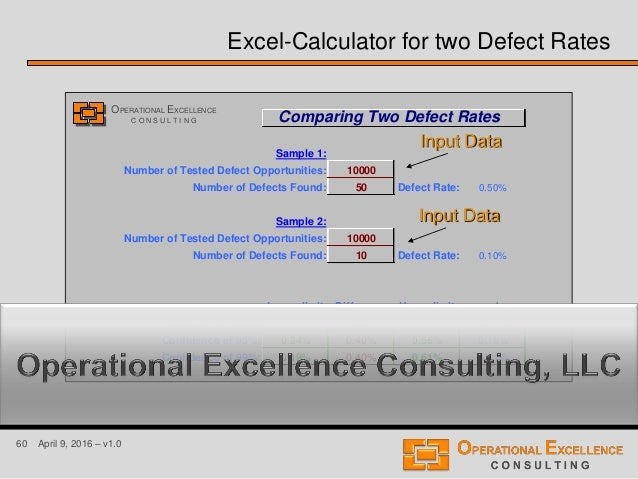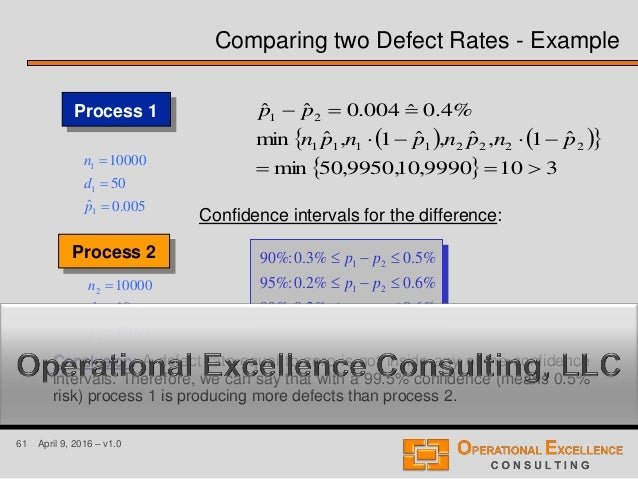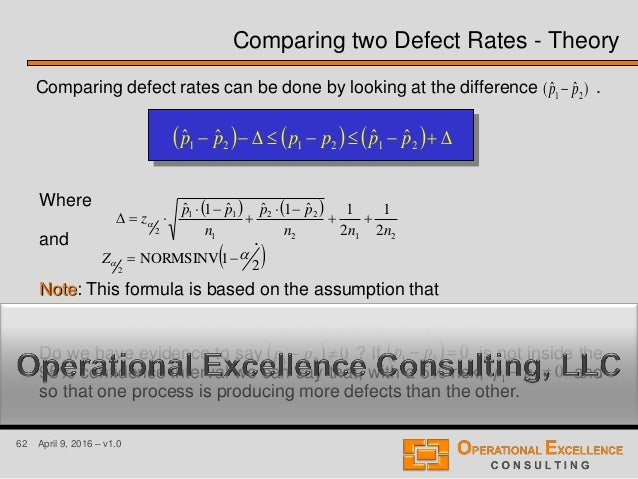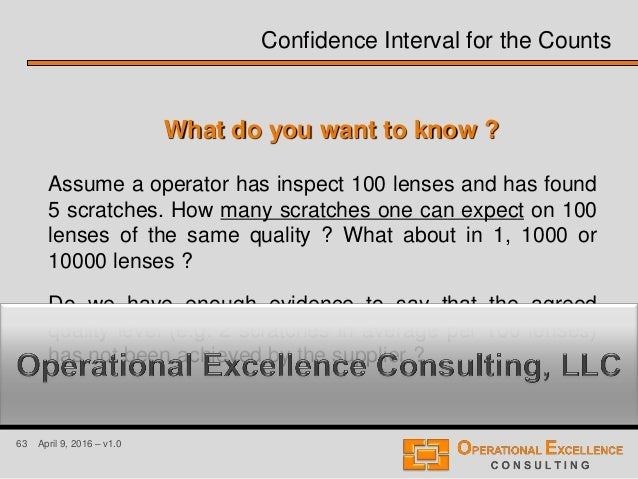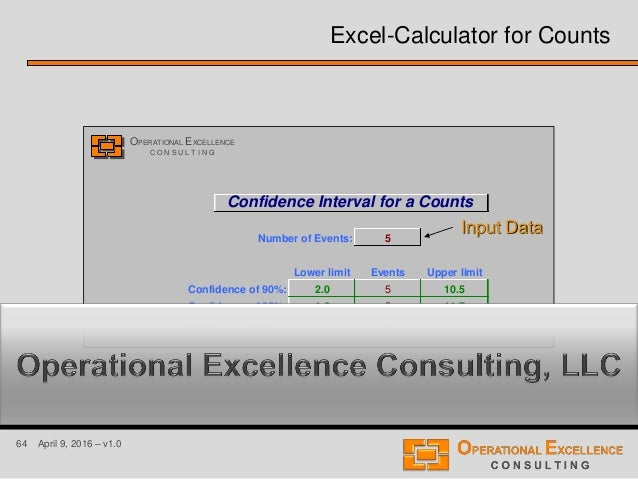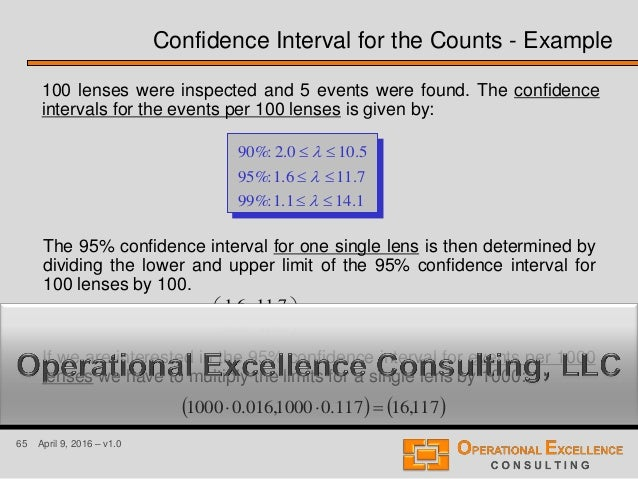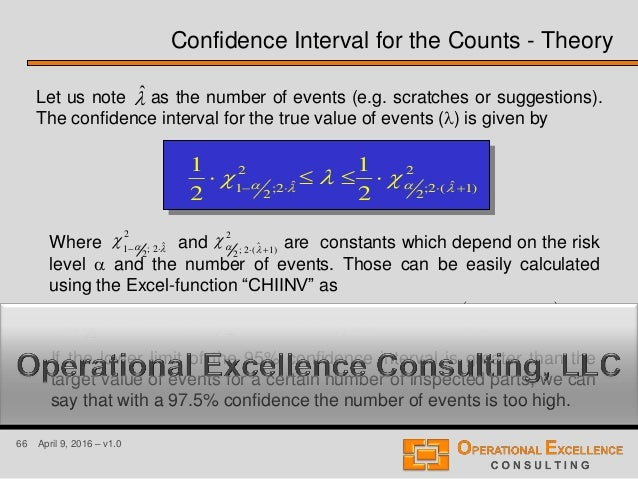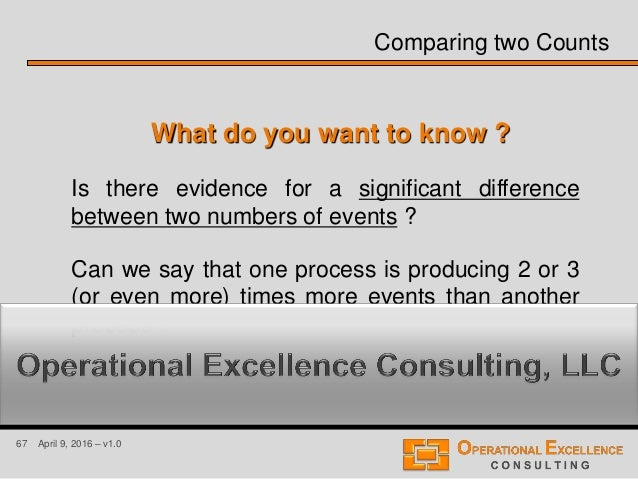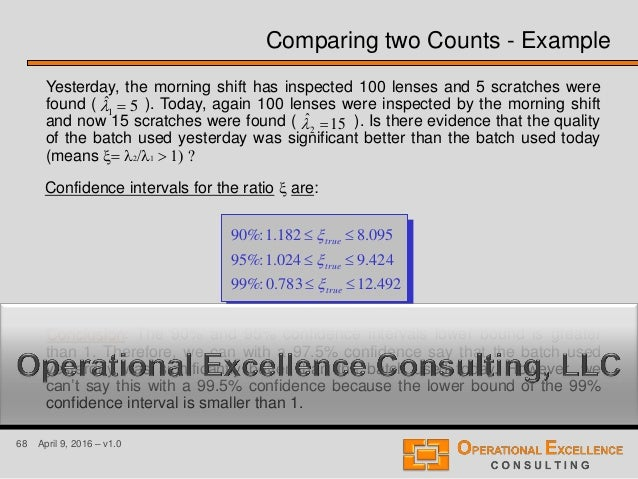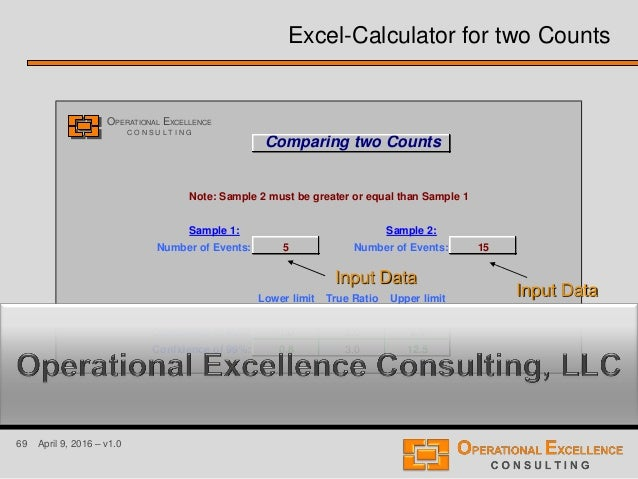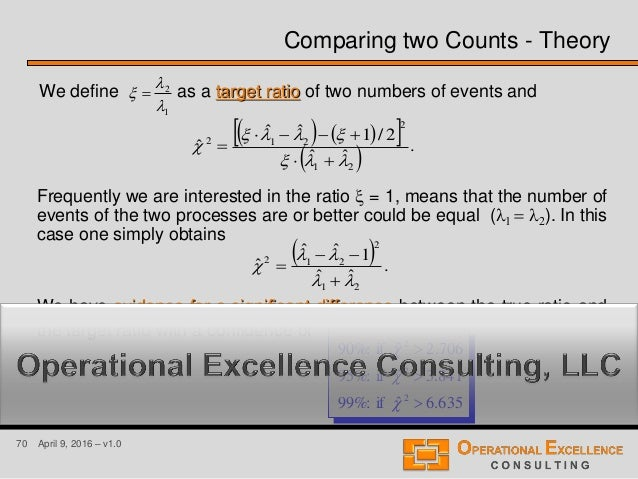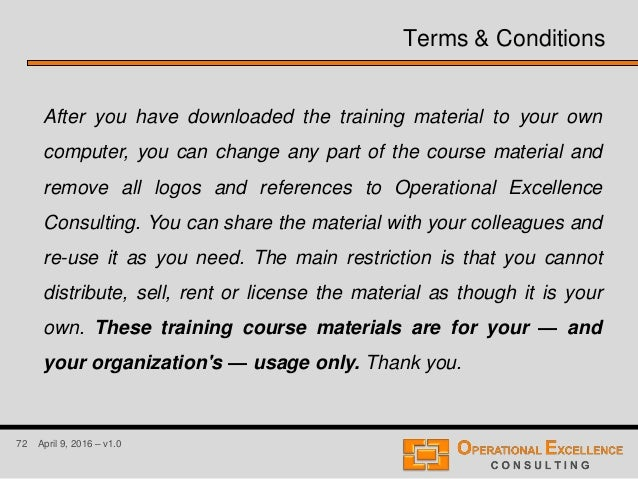Upcoming SlideShare
×

# Six Sigma Confidence Interval Analysis (CIA) Training Module

The Six Sigma Confidence Interval Analysis (CIA) Training Module v1.0 includes:

1. MS PowerPoint Presentation including 72 slides covering theory and examples of Confidence Interval Analysis and Hypothesis Testing for CIA for one Mean Value, Comparison of two Mean Values, Comparison of a Paired Data Sets, CIA for one Standard Deviation, Comparison of two Standard Deviations, CIA for Capability Indices, CIA for one Defect Rate, Comparison of two Defect Rates, CIA for one Count, and Comparison of two Counts.

2. MS Excel Six Sigma Confidence Interval Analysis Calculator making it really easy to calculate Confidence Intervals (mean value, standard deviation, capability indices, defect rate, count) and perform a Comparison of two Statistics (mean values, standard deviations, defect rates, counts).

• Full Name
Comment goes here.

Are you sure you want to Yes No• Be the first to comment

### Six Sigma Confidence Interval Analysis (CIA) Training Module

1. 1. 1 April 9, 2016 – v1.0 Six Sigma Confidence Interval Analysis by Operational Excellence Consulting LLC
2. 2. 2 April 9, 2016 – v1.0 Why should we use Intervals ? Sometimes these questions tend to be of two types: “How has something been performing in the past ?” and “How will something perform in the future ?” It is important to recognise that the manner in which we collect and analyse data dictates the quality of our results. Unfortunately, it is often assumed that the estimated parameter based on a sample is the “true” value. This is not accurate !!! Therefore, the natural question to ask has to be: “How good is the estimation one obtains from a sample ?” To answer this question, one must turn to the method of interval estimation. This technique extend a estimation of a product/process parameter to a so-called confidence interval of values that contain the “true” parameter with a pre-defined confidence or probability.
3. 3. 3 April 9, 2016 – v1.0 Data-Based Decision Making 1. Plan: What do you want to know ? How do you want to see what it is that you need to know ? What type of tool will generate what it is that you need to see ? What type of data is required to the selected tool ? Where can you get the required type of data ? 2. Collect Data 3. Analyse Data 4. Interpret Data
4. 4. 4 April 9, 2016 – v1.0 Introduction to Confidence Interval Analysis This module will help improve decision making and communication when data are used. The opportunity for improvement exists because: • Sometimes we don’t recognise that there is uncertainty in our data. • Sometimes we want to report an appropriate interval but the calculation methods are cumbersome or confusing. • Sometimes we attempt to make statements about our uncertainty but do so improperly [like reporting a (± 3 std.dev.) or ± (max. - min.)/2 interval when some other type of interval is more appropriate].
5. 5. 5 April 9, 2016 – v1.0 Definition of a Confidence Interval A 90/95/99% confidence interval for a population parameter (e.g. mean/average, standard deviation, cp, cpk, defect rate, etc.) is an interval around the estimated parameter that has a 90/95/99% chance of capturing the true value of the parameter. The value  is called the risk level of the decision. With a confidence of 90/95/99% we have a 10/5/1% risk which means . 5.0% 2.5% 0.5% 5.0% 2.5% 0.5% 99% 95% 90% xestimation
6. 6. 6 April 9, 2016 – v1.0 Assumptions for Confidence Interval Analysis There are three fundamental assumptions to all of the following intervals: • Control • The data comes from a single, stable distribution. This assumption should be verified by plotting the data in time sequence (trend or control chart). Being in control is important from two standpoints: • The descriptive statistics lose meaning if the process is significantly out of control. • A state of control provides the ability to predict future process behaviour.
7. 7. 7 April 9, 2016 – v1.0 • Randomness • The sample is a random sample. The question of a sample being random should be addressed before the data is collected. • Normality • The assumption of fair normality is most important when dealing with confidence intervals for standard deviations or Process Capability Indices as cp and cpk. It can be assessed through the use of histograms (as evidenced by a symmetric, “bell-shaped” curve) or some statistical methods like the Kolmogorov-Smirnov test or the Normality Plot. Assumptions for Confidence Interval Analysis
8. 8. 8 April 9, 2016 – v1.0 What do you want to know ? We can take a sample in production and estimate the mean value or average of a product or process, but what is the “real” mean value ? Can we say that there is evidence that the estimated mean value is different from a pre-defined target value T ? Confidence Interval for a Mean Value
9. 9. 9 April 9, 2016 – v1.0 03.7 02.57 10 1 1 1    estim estim s x n Sample 1. 84.9 12.61 50 2 2 2    estim estim s x n Sample 2. Confidence Interval for a Mean Value Which one is the real mean value ? What can we conclude about the real mean value based on the estimated mean value ? Is the sample size sufficiently large ?
10. 10. 10 April 9, 2016 – v1.0 12.61 50 00.58...33.7300.72 ...21      n xxx x n n= 50 84.9 150 )12.6158(...)12.6133.73()12.6172( 1 )(...)()( 222 22 2 2 1        n xxxxxx s n 72.00 73.33 65.33 58.00 59.00 88.83 73.67 62.50 51.17 55.67 52.33 55.83 55.00 77.17 53.33 58.00 Confidence Interval for a Mean Value - Example m 0.0 4.8 9.6 14.4 19.2 24.0 0.00 6.80 13.60 20.40 27.20 34.00C E L L F R E Q U E N C Y P E R C E N T CELL BOUNDARY 44.8 53.6 62.4 71.2 80.0 88.8 Fitted curve is a Normal. K-S test: 0.326. Lack of fit is not significant.
11. 11. 11 April 9, 2016 – v1.0 Confidence Interval for a Mean or Average Number of Observations 50 Mean or Average 61.12 Standard Deviation 9.840 Lower limit Average Upper limit ± D t(/2) Confidence of 90%: 58.79 61.12 63.45 2.33 1.677 Confidence of 95%: 58.32 61.12 63.92 2.80 2.010 Confidence of 99%: 57.39 61.12 64.85 3.73 2.680 OPERATIONAL EXCELLENCE C O N S U L T I N G Excel-Calculator for a Mean Value Input Data
12. 12. 12 April 9, 2016 – v1.0 Can we decide with a confidence of 95(99)% (means a 5(1)% risk) that the true mean value of the process is better than 58.50% ? Conclusion: There is a 5 % risk that the true process mean value is lower than 58.79%. However, there is a 95% confidence that the true process mean value is greater than 58.79% and so of course also greater than the target value 58.50%. 57.39 58.32 58.79 63.45 63.92 64.8561.12 Confidence Interval for a Mean Value - Example 85.6439.57:%99 92.6332.58:%95 45.6379.58:%90    true true true x x x 58.50 57.39 64.8557.39 64.8557.39
13. 13. 13 April 9, 2016 – v1.0 n s txx n s tx estimtrueestim  22  where is a constant that depends on the risk level  and the number of observations n in the sample, it can be easily calculated using the Excel-function “TINV” as =TINV(;n-1)t  t  Confidence Interval for the Mean Value - Theory estimxestimx Small variation higherconfidence Large variation lowerconfidence
14. 14. 14 April 9, 2016 – v1.0 What do you want to know ? Can we say that two samples are not taken from the same process ? Does evidence for a significant difference exist between two processes (e.g. current/better), two test groups, or two persons performing the same work/measurements ? Comparing two Mean Values
15. 15. 15 April 9, 2016 – v1.0 03.7 02.57 10 1 1 1    s x n estim Sample 1. 84.9 12.61 50 2 2 2    s x n estim Sample 2. or 03.7 02.57 10 1 1 1    s x n estim Sample 1. 84.9 12.61 50 2 2 2    s x n estim Sample 2. ? Comparing two Mean Values or ? or ?
16. 16. 16 April 9, 2016 – v1.0 (for equal standard deviations) Sample 1: Number of Observations 10 Confidence of 90%: 52.94 61.10 Mean or Average 57.02 Confidence of 95%: 51.99 62.05 Standard Deviation 7.030 Confidence of 99%: 49.80 64.24 Sample 2: Number of Observations 50 Confidence of 90%: 58.79 63.45 Mean or Average 61.12 Confidence of 95%: 58.32 63.92 Standard Deviation 9.840 Confidence of 99%: 57.39 64.85 Lower limit Difference Upper limit ± D Confidence of 90%: -1.38 4.10 9.58 5.48 Confidence of 95%: -2.46 4.10 10.66 6.56 Confidence of 99%: -4.63 4.10 12.83 8.73 Pooled Std. Dev.= 9.46 Comparing two MeansOPERATIONAL EXCELLENCE C O N S U L T I N G Excel-Calculator for two Mean Values with s1 = s2 Input Data Input Data
17. 17. 17 April 9, 2016 – v1.0 Process 1 03.7 02.57 10 1 1 1    s x n estim 95% confidence interval: Process 2 84.9 12.61 50 2 2 2    s x n estim 95% confidence interval: 05.6299.51  truex 92.6332.58  truex 50 55 60 65 Is there evidence for a significant process improvement ? Comparing two Mean Values - Example
18. 18. 18 April 9, 2016 – v1.0 84.9 12.61 50 2 2 2    s x n estim 03.7 02.57 10 1 1 1    s x n estim 63.483.12:%99 46.266.10:%95 38.158.9:%90 D D D true true true Conclusion: Because zero is inside all confidence intervals we have not enough evidence to say that there is a significant difference between the two processes. Confidence intervals for the difference of two means: Comparing two Mean Values with s1 = s2 - Example Process 2 Process 1
19. 19. 19 April 9, 2016 – v1.0 Comparing two Means (for unequal standard deviations) Sample 1: Number of Observations 10 Confidence of 90%: 52.94 61.10 Mean or Average 57.02 Confidence of 95%: 51.99 62.05 Standard Deviation 7.030 Confidence of 99%: 49.80 64.24 Sample 2: Number of Observations 50 Confidence of 90%: 58.79 63.45 Mean or Average 61.12 Confidence of 95%: 58.32 63.92 Standard Deviation 9.840 Confidence of 99%: 57.39 64.85 Lower limit Difference Upper limit ± D Confidence of 90%: -0.48 4.10 8.68 4.58 Confidence of 95%: -1.46 4.10 9.66 5.56 Confidence of 99%: -3.56 4.10 11.76 7.66 Degrees of Freedom: 16.00 OPERATIONAL EXCELLENCE C O N S U L T I N G Excel-Calculator for two Mean Values with s1  s2 Input Data Input Data
20. 20. 20 April 9, 2016 – v1.0 84.9 12.61 50 2 2 2    s x n estim 03.7 02.57 10 1 1 1    s x n estim 56.376.11:%99 46.166.9:%95 48.068.8:%90 D D D true true true Conclusion: Because zero is inside all confidence intervals we have not enough evidence to say that there is a significant difference between the two processes. Confidence intervals for the difference of two means: Comparing two Mean Values with s1  s2 - Example Process 2 Process 1
21. 21. 21 April 9, 2016 – v1.0     .if, 2 or 2 11 21 2 2 2 1 21 2 22 2 11 nn ss S nn snsn S Pp       To combine two standard deviations we have to calculate the pooled standard deviation: Confidence interval for the difference isDtrue true truex x 1 2 21 2 21 2 1111 nn St nn St pestimtruepestim DDD  where tcan be calculated as  .2;TINV 212  nnt  The question is now, do we have evidence to say ? If zero is not inside the 95% confidence interval we can say with a 95% confidence or a 5% risk that the two process are different. 0Dtrue Comparing two Mean Values with s1 = s2 - Theory
22. 22. 22 April 9, 2016 – v1.0                            11 2 2 2 2 2 1 2 1 2 1 2 2 2 2 1 2 1 n ns n ns n s n s  To compare two mean values with different standard deviations we have to calculate a “pooled” degree of freedom: Confidence interval for the difference is21 truetruetrue xx D 2 2 2 1 2 1 2 2 2 2 1 2 1 2 n s n s t n s n s t estimtrueestim DDD  where tcan be calculated as  .;TINV2  t The question is now, do we have evidence to say ? If zero is not inside the 95% confidence interval we can say with a 95% confidence or a 5% risk that the two process are different. 0Dtrue Comparing two Mean Values with s1  s2 - Theory This value will not be nessarily be an integer. If it is not, we round it down to the nearest integer.
23. 23. 23 April 9, 2016 – v1.0 What do you want to know ? Can we say that two data sets are not taken or generated by the same process or method ? Does evidence for a significant difference exist between two methods (e.g. current/better), two test groups, or two persons performing the same work/measurements ? Comparing Mean Values for Paired Data
24. 24. 24 April 9, 2016 – v1.0 For example: We can compare measurement results obtained for example from two different persons measuring the same products. Data has to be collected pairwise so that one pair contains measurement results from the same products. Pair 1 2 3 ... n Person 1 X1 X2 X3 ... Xn Person 2 Y1 Y2 Y3 ... Yn Results D1 D2 D3 ... D n       1 ... ... ,...3,2,1, 21 21  DDDDDD  DDD D D D n s n niYX estimnestimestim n estim iii (differences within the pairs) (average of differences within the pairs) (standard deviation of differences) Define: Comparing Mean Values for Paired Data
25. 25. 25 April 9, 2016 – v1.0 0233.00013.0:%99 0196.00024.0:%95 0180.00040.0:%90 D D D true true true Pair 1 2 3 4 5 6 7 8 9 10 Person 1 13.21 13.15 13.18 13.20 13.19 13.18 13.22 13.20 13.19 13.21 Person 2 13.19 13.14 13.18 13.17 13.18 13.18 13.20 13.21 13.17 13.20 D 0.02 0.01 0.00 0.03 0.01 0.00 0.02 -0.01 0.02 0.01 0120.0011.0 D Dsestim Confidence intervals for the Differences: Conclusion: Zero is not included in the 95% confidence interval. Therefore, we can say, with a 5% risk, that the two persons are measuring in significantly different ways. However, zero is included in the 99% confidence interval, thus with a confidence of 99% we can not say that the persons are doing the measurement in different way. Comparing Mean Values for Paired Data - Example
26. 26. 26 April 9, 2016 – v1.0 Comparing Paired Data Number of Pairs 10 Average of Differences 0.011 Standard Deviation of Differences 0.012 Lower limit Average Upper limit ± D t(α/2) Confidence of 90%: 0.004 0.011 0.018 0.007 1.833 Confidence of 95%: 0.002 0.011 0.020 0.009 2.262 Confidence of 99%: -0.001 0.011 0.023 0.012 3.250 OPERATIONAL EXCELLENCE C O N S U L T I N G Excel-Calculator for Paired Data Input Data
27. 27. 27 April 9, 2016 – v1.0 n s t n s t estimtrueestim DD DDD 22  where tcan be calculated as  1;TINV2  nt  If zero is not inside the 95% confidence interval we can say (with a 5% risk) that the two persons are performing the measurement process in significantly different ways or as exist at least some reason why they are getting significantly different measurement results. Comparing Mean Values for Paired Data - Theory
28. 28. 28 April 9, 2016 – v1.0 Confidence Interval for a Standard Deviation What do you want to know ? We can take a sample in production and estimate the standard deviation, but what is the real standard deviation of the process ? Can we say that the process standard deviation is small enough compared to a given tolerance interval ?
29. 29. 29 April 9, 2016 – v1.0 Confidence Interval for a Standard Deviation 03.7 02.57 10 1 1 1    estim estim s x n Sample 1. 84.9 12.61 50 2 2 2    estim estim s x n Sample 2. Which one is the real standard deviation ? What can we conclude about the real standard deviation based on the estimated standard deviation ? Is the sample size sufficiently large ?
30. 30. 30 April 9, 2016 – v1.0 Confidence Interval for a Standard Deviation Number of Observations 50 Standard Deviation 9.840 Lower limit Std. Dev. Upper limit Confidence of 90%: 8.457 9.840 11.825 Confidence of 95%: 8.220 9.840 12.262 Confidence of 99%: 7.788 9.840 13.195 OPERATIONAL EXCELLENCE C O N S U L T I N G Excel-Calculator for a Standard Deviation Input Data
31. 31. 31 April 9, 2016 – v1.0 Confidence Interval for a Standard Deviation - Example Can we say that our sample gives us enough evidence to say that the process standard deviation is smaller than the target standard deviation equal to s = 8 ? 84.9 50   estims n 20.1379.7:%99 26.1222.8:%95 82.1146.8:%90    true true true s s s Conclusion: The target value of s = 8 is only inside the 99% confidence interval. Therefore, we can say with 97.5% confidence (means 2.5% risk) that the true standard deviation is higher than the target value. However, we can’t say so with a 99.5% confidence, because the lower bound of the 99% confidence interval is smaller than 8. Sample: Confidence intervals:
32. 32. 32 April 9, 2016 – v1.0 Confidence Interval for a Standard Deviation - Theory 2 2 1 2 2 11        n ss n s estimtrueestim where and are constants depending on the risk level  and the number of observations n in the sample. Those can easily be calculated using the Excel-function “CHIINV” as and If the target standard deviation is higher than the upper limit of the 90% confidence interval we have only a 5% risk that the real process standard deviation is not small enough.  1;2CHIINV2 2  n  1;21CHIINV2 21  n  2 2 2 21  where and are constants depending on the risk level  and the number of observations n in the sample. Those can easily be calculated using the Excel-function “CHIINV” as and If the target standard deviation is higher than the upper limit of the 90% confidence interval we have only a 5% risk that the real process standard deviation is not small enough. 2 22 2where and are constants depending on the risk level  and the number of observations n in the sample. Those can easily be calculated using the Excel-function “CHIINV” as and If the target standard deviation is higher than the upper limit of the 90% confidence interval we have only a 5% risk that the real process standard deviation is not small enough.
33. 33. 33 April 9, 2016 – v1.0 Comparing two Standard Deviations Is there evidence for a significant process improvement with respect to a decreased standard deviation, i.e. is there a significant difference between the standard deviations of the two processes ? What do you want to know ?
34. 34. 34 April 9, 2016 – v1.0 Comparing two Standard Deviations 03.7 10 1 1   s n Sample 1. 84.9 50 2 2   s n Sample 2. or 03.7 10 1 1   s n Sample 1. 84.9 50 2 2   s n Sample 2. ? or ??
35. 35. 35 April 9, 2016 – v1.0 Comparing two Standard Deviations Sample 1: Confidence of 90%: 5.13 11.57 Number of Observations 10 Confidence of 95%: 4.84 12.83 Standard Deviation 7.030 Confidence of 99%: 4.34 16.01 Sample 2: Confidence of 90%: 8.46 11.82 Number of Observations 50 Confidence of 95%: 8.22 12.26 Standard Deviation 9.840 Confidence of 99%: 7.79 13.20 Lower limit Ratio Percentage Upper limit Confidence of 90%: 0.50 0.71 40.0 1.20 Confidence of 95%: 0.46 0.71 40.0 1.33 Confidence of 99%: 0.41 0.71 40.0 1.67 OPERATIONAL EXCELLENCE C O N S U L T I N G Excel-Calculator for two Standard Deviations Input Data Input Data
36. 36. 36 April 9, 2016 – v1.0 Comparing two Standard Deviations - Example Is there evidence for a significant process improvement ? 03.7 10 1 1   s n 95% confidence interval for the standard deviation: Process 2 84.9 50 2 2   s n 95% confidence interval for the standard deviation: 83.1284.4  trues 26.1222.8  trues 4 86 1210 Process 1
37. 37. 37 April 9, 2016 – v1.0 84.9 50 2 2   s n 03.7 10 1 1   s n 714.0 2 1  estim estim estim s s  Confidence intervals for the ratio :true 67.141.0:%99 33.146.0:%95 20.150.0:%90    true true true    Conclusion: Because is inside all confidence intervals we have not enough evidence to conclude that there is a significant difference between the standard deviations of the two processes. Process 2 1true Comparing two Standard Deviations - Example Process 1 Confidence intervals for the ratio : Conclusion: Because is inside all confidence intervals we have not enough evidence to conclude that there is a significant difference between the standard deviations of the two processes.
38. 38. 38 April 9, 2016 – v1.0 We simply compare with by looking at their ratio Confidence interval for the ratio : 1estims 2estims Comparing two Standard Deviations - Theory 2 1 estim estim estin s s  2 1 true true true s s           estimtrueestim F F  2 1 1 Where F1 and F2 are constants which depend on the risk level  and the number n of observations in the samples. Those can be easily calculated using the Excel-function “FINV” as F1 = FINV(/2;n1–1;n2–1) and F2 = FINV(/2;n2– 1;n1–1). Do we have evidence to say ? If is not inside the 95% confidence interval, then we can say that with a 95% confidence or a 5% risk the standard deviations of the two process are significantly different. 1true 1true
39. 39. 39 April 9, 2016 – v1.0 Confidence Interval for the and The estimated and capability indices are for example based on 25 subgroups with 5 observations in each subgroup, i.e. 125 measurements were taken. What are the real capability indices ? Can we say that our process is not capable in other words, can we say that is smaller than 1.33 ? What do you want to know ? pkcˆ pkcˆ pcˆ pcˆ pkcˆ
40. 40. 40 April 9, 2016 – v1.0 A Measure of Process Capability  For any critical characteristic the Capability Index (a “hat” (^) will denote an estimated quantity), also known as the design margin, is quantified by : ST p s LSLUSL c    6 - )variationprocess(or totalCapabilityProcess erance)design tol(orion WidthSpecificat ˆ pcˆ
41. 41. 41 April 9, 2016 – v1.0 The Capability Index pcˆ average = nominal value or target LSL USLLCL UCL 1.00 < cp < 1.33 average + 3*sigma average - 3*sigma
42. 42. 42 April 9, 2016 – v1.0 A Measure of Process Performance  is the Capability Index adjusted for k, which considers actual operation of the process and takes into account any difference between the design nominal and the actual process mean value x-bar. ) 3 - , 3 - (minˆ STST pk s LSLx s xUSL c   pkcˆ
43. 43. 43 April 9, 2016 – v1.0 The Capability Index pkcˆ nominal value LSL USLLCL UCL average 1.33 < cp < 2.00, but 1.00 < cpk < 1.33 average - 3*sigma average + 3*sigma
44. 44. 44 April 9, 2016 – v1.0 Confidence Interval for the and Mean value = Nominal value or Target LSL USL = ? Nominal value LSL USL Mean value ? pcˆ pcˆ pkcˆ pkcˆpkcˆ Uncertainty in standard deviation Uncertainty in mean value
45. 45. 45 April 9, 2016 – v1.0 Number of Observations: 90 Cp: 5.00 Cpk: 2.30 PPM: 0 Lower limit Cp Upper limit ± D Confidence of 90%: 4.38 5.00 5.62 0.62 Confidence of 95%: 4.27 5.00 5.73 0.73 Confidence of 99%: 4.03 5.00 5.97 0.97 Lower limit Cpk Upper limit ± D Confidence of 90%: 2.01 2.30 2.59 0.29 Confidence of 95%: 1.96 2.30 2.64 0.34 Confidence of 99%: 1.85 2.30 2.75 0.45 Confidence Interval for Capability Indices OPERATIONAL EXCELLENCE C O N S U L T I N G Excel-Calculator for Capability Indices Input Data
46. 46. 46 April 9, 2016 – v1.0 Sample 30.2ˆ 00.5ˆ 90518    pk p c c m 97.503.4:%99 73.527.4:%95 62.538.4:%90    p p p c c c 75.285.1:%99 64.296.1:%95 59.201.2:%90    pk pk pk c c c Conclusion: With a risk of less than 0.5% we can say that the process is capable. The lower limit of every cp -confidence interval is greater than 1.33 and the lower limit of every cpk - confidence interval is greater than 1.33. nominal value LSL USLLCL UCL averageAverage - 3*s Average + 3*s Confidence Interval for the and - Examplepcˆ pkcˆ
47. 47. 47 April 9, 2016 – v1.0    12 ˆ ˆ 12 ˆ ˆ 22     m c zcc m c zc p pp p p  Where m = n · N is the number of measurements (n is the number of products/ measurements in each subgroup and N is the number of subgroups) and is a constant that depends on the risk level . This constant can be easily calculated using the Excel-function “NORMSINV” as . If for example the 90% confidence interval includes the value 1.33 we can not make any decision about the process capability. If the upper limit of the 90% confidence interval is < 1.33 we can say, with a 5% risk, that the process is not capable. If the lower limit of the 90% confidence interval is  1.33 we can say, with a 5% risk, that the process is capable. z 2 )2-NORMSINV(12  z pcˆConfidence Interval for the - Theory
48. 48. 48 April 9, 2016 – v1.0 pcˆConfidence Interval for the 95% confidence interval 0.0000 0.2000 0.4000 0.6000 0.8000 1.0000 1.2000 1.4000 1.6000 0 100 200 300 400 500 600 m=N*n delta cp=0.5 cp=1.0 cp=1.5 cp=2.0
49. 49. 49 April 9, 2016 – v1.0     mm c zcc mm c zc pk pkpk pk pk         9 1 12 ˆ ˆ 9 1 12 ˆ ˆ 2 2 2 2  Where m = n · N is the number of measurements (n is the number of products/ measurements in each subgroup and N is the number of subgroups) and is a constant that depends on the risk level . This constant can easily be calculated using the Ecxel-function “NORMSINV” as . If the upper limit of the 90% confidence interval is < 1.33 we can say, with a 5% risk, that the process is not capable (location of distribution is wrong). If the lower limit of the 90% confidence interval is 1.33 we can say, with a 5% risk, that the process is capable. z 2 )2-NORMSINV(12  z pkcˆConfidence Interval for the - Theory
50. 50. 50 April 9, 2016 – v1.0 Confidence Interval for the 95% confidence interval 0.0000 0.2000 0.4000 0.6000 0.8000 1.0000 1.2000 1.4000 1.6000 0 100 200 300 400 500 600 m=N*n delta cpk=0.5 cpk=1.0 cpk=1.5 cpk=2.0 pkcˆ
51. 51. 51 April 9, 2016 – v1.0 We can take a sample in production or in pre- production and estimate the defect rate ( ) of a process, but what is the real defect rate (p) ? Do we have enough evidence to say that a defined target is achieved ? What do you want to know ? pˆ Confidence Interval for Defect Rates
52. 52. 52 April 9, 2016 – v1.0 A new IC (160 solder joints per IC) shall be used for a new product. The product development team decided to purchase 100 ICs and test they solderability. An experiment was organised in pre-production and during inspection two (2) poor solder joints were identified. This results in a estimated defect rate for one solder joint of %01.0% 100160 2 100% partsof#ies/partoppurtunitdefectof# defectsof# 100ˆ     p Which is equal to a PPM level of 12510 %100 ratedefect 6 PPM Confidence Interval for Defect Rates - Example Is this evidence enough to conclude that with a confidence of 95% the defect rate for this part will be less than 250 PPM ?
53. 53. 53 April 9, 2016 – v1.0 Number of Tested Defect Opportunities: 16000 Number of Defects Found: 2 Lower limit p (%) Upper limit Confidence of 90%: 0.00% 0.01% 0.04% Confidence of 95%: 0.00% 0.01% 0.05% Confidence of 99%: 0.00% 0.01% 0.06% Lower limit p (DPM) Upper limit Confidence of 90%: 22 125 393 Confidence of 95%: 15 125 451 Confidence of 99%: 6 125 579 Confidence Interval for a Defect Rate OPERATIONAL EXCELLENCE C O N S U L T I N G Excel-Calculator for Defect Rates Input Data
54. 54. 54 April 9, 2016 – v1.0 Sample: PPM p d n 125=ˆ %01.0ˆ 2 00016    Note, that the number of defect opportunities is given by jointssolder00016 component jointssolder 160components100  Conclusion: With a 5% risk the true defect rate (in PPM) will be above 393 PPM. However, with a confidence of 90% the true defect rate will be in the interval [22, 393] PPM. For a target value of for example less than 250 PPM we can’t make any conclusion. If the range of possible defect rates is too larger we just have to place, solder and inspect more ICs components. %06.0%00.0:%99 %05.0%00.0:%95 %04.0%00.0:%90    p p p PPMpPPM PPMpPPM PPMpPPM 5796:%99 45115:%95 39322:%90    Confidence Interval for Defect Rates - Example
55. 55. 55 April 9, 2016 – v1.0 Sample: PPMp d n 0=%00.0ˆ 0 00016    Conclusion: With a 5% risk the true defect rate (in PPM) will be greater than 187 PPM. However, with a confidence of 95% the true defect rate will be between 0 PPM and 187 PPM. For a target value less than 250 PPM we do have a confidence of about 95% that the solderability of the IC’s is good enough. However, the upper limit of the 99% confidence interval is greater than the target value and so we have a risk higher than 1% but lower than 5% that the solderability will not be good enough. %03.0%0:%99 %02.0%0:%95 %01.0%0:%90    p p p PPMpPPM PPMpPPM PPMpPPM 2880:%99 1870:%95 1440:%90    Confidence Interval for Defect Rates - Example
56. 56. 56 April 9, 2016 – v1.0 In cases where the estimated defect rate is equal to zero , the natural lower limit of the confidence interval is zero. Thus, there exists no risk that the true value of the defect rate p is smaller than zero. Therefore, the upper limit of the confidence interval has to be calculated in such way that with a risk of 100·% the true value of the parameter p is greater than the upper limit.  0ˆ p %100%0 2 2 Fn F p   Where n is the number of tested defect opportunities and . nF  2;2;FINV2  Confidence Interval for Defect Rates - Theory
57. 57. 57 April 9, 2016 – v1.0 In cases where the estimated defect rate is greater than zero, means , a two sided confidence interval is calculated by 0ˆ p % )1( )1( 100% )1( 100 2 2 1 Fddn Fd p Fdnd d      where n is the number of tested defect opportunities (sample size) and d the number of defects found. The values and can be easily calculated using the Excel-function “FINV” as and 21 FF )2);1(2;2/(1 ddnFINVF   ))(2);1(2;2/(2 dndFINVF   If the lower bound of 90% confidence interval is greater than the target value for the defect rate we can say, with a 5% risk, that the defect rate is too high compared with the target value. Confidence Interval for Defect Rates - Theory
58. 58. 58 April 9, 2016 – v1.0 Comparing two Defect Rates What do you want to know ? If we compare the defect rates of two processes (e.g. two production lines) can we say that one process is producing more defects than the other ? Can we say that there is evidence for a significant process improvement with respect to the defect rates ?
59. 59. 59 April 9, 2016 – v1.0 Comparing two Defect Rates - Example Process 2 PPM0005ˆ%5.0ˆ005.0ˆ 50 00010 1 1 1 1 1    n d p d n PPM0001ˆ%1.0ˆ001.0ˆ 10 00010 1 1 2 2 2    n d p d n 95% confidence interval for the defect rate: 95% confidence interval for the defect rate: PPM5876PPM7133 %66.0%37.0 1 1   p p PPM8381PPM480 %18.0%05.0 2 2   p p PPM Process 1
60. 60. 60 April 9, 2016 – v1.0 Comparing Two Defect Rates Sample 1: Number of Tested Defect Opportunities: 10000 Number of Defects Found: 50 Defect Rate: 0.50% Sample 2: Number of Tested Defect Opportunities: 10000 Number of Defects Found: 10 Defect Rate: 0.10% Lower limit Difference Upper limit ± D Confidence of 90%: 0.26% 0.40% 0.54% 0.14% Confidence of 95%: 0.24% 0.40% 0.56% 0.16% Confidence of 99%: 0.19% 0.40% 0.61% 0.21% OPERATIONAL EXCELLENCE C O N S U L T I N G Excel-Calculator for two Defect Rates Input Data Input Data
61. 61. 61 April 9, 2016 – v1.0 005.0ˆ 50 10000 1 1 1    p d n 001.0ˆ 10 10000 2 2 2    p d n        3109990,10,9950,50min ˆ1,ˆ,ˆ1,ˆmin %4.0ˆ004.0ˆˆ 22221111 21    pnpnpnpn pp Confidence intervals for the difference: %6.0%2.0:%99 %6.0%2.0:%95 %5.0%3.0:%90 21 21 21    pp pp pp Conclusion: A defect rate equal to zero is not inside any of the confidence intervals. Therefore, we can say that with a 99.5% confidence (means 0.5% risk) process 1 is producing more defects than process 2. Comparing two Defect Rates - Example Process 1 Process 2
62. 62. 62 April 9, 2016 – v1.0 Comparing defect rates can be done by looking at the difference .)ˆˆ( 21 pp        DD 212121 ˆˆˆˆ pppppp Where and .     212 22 1 11 2 2 1 2 1ˆ1ˆˆ1ˆ nnn pp n pp z     D   2 1NORMSINV 2  Z Note: This formula is based on the assumption that      3ˆ1,ˆ,ˆ1,ˆmin 22221111  pnpnpnpn Do we have evidence to say ? If is not inside the 95% confidence interval we can say that, with a 5% risk, and so that one process is producing more defects than the other.   021  pp 021  pp   021  pp Comparing two Defect Rates - Theory
63. 63. 63 April 9, 2016 – v1.0 Confidence Interval for the Counts What do you want to know ? Assume a operator has inspect 100 lenses and has found 5 scratches. How many scratches one can expect on 100 lenses of the same quality ? What about in 1, 1000 or 10000 lenses ? Do we have enough evidence to say that the agreed quality level (e.g. 2 scratches in average per 100 lenses) has not been achieved by the supplier ?
64. 64. 64 April 9, 2016 – v1.0 Confidence Interval for a Counts Number of Events: 5 Lower limit Events Upper limit Confidence of 90%: 2.0 5 10.5 Confidence of 95%: 1.6 5 11.7 Confidence of 99%: 1.1 5 14.1 OPERATIONAL EXCELLENCE C O N S U L T I N G Excel-Calculator for Counts Input Data
65. 65. 65 April 9, 2016 – v1.0 Confidence Interval for the Counts - Example 100 lenses were inspected and 5 events were found. The confidence intervals for the events per 100 lenses is given by: 1.141.1:%99 7.116.1:%95 5.100.2:%90       The 95% confidence interval for one single lens is then determined by dividing the lower and upper limit of the 95% confidence interval for 100 lenses by 100.  117.0,016.0 100 7.11 , 100 6.1       If we are interested in the 95% confidence interval for events per 1000 lenses we have to multiply the limits for a single lens by 1000:    117,16117.01000,016.01000 
66. 66. 66 April 9, 2016 – v1.0 Let us note as the number of events (e.g. scratches or suggestions). The confidence interval for the true value of events () is given by ˆ 2 )1ˆ(2; 2 2 ˆ2; 2 1 2 1 2 1     Where and are constants which depend on the risk level  and the number of events. Those can be easily calculated using the Excel-function “CHIINV” as and   1 2 2 2  ;   2 2 1) 2 ; (      ˆ2; 2 1CHIINV2 ˆ2; 2 1   )1ˆ(2; 2 CHIINV2 )1ˆ(2; 2    If the lower limit of the 95% confidence interval is greater than the target value of events for a certain number of inspected parts, we can say that with a 97.5% confidence the number of events is too high. Confidence Interval for the Counts - Theory
67. 67. 67 April 9, 2016 – v1.0 Comparing two Counts What do you want to know ? Is there evidence for a significant difference between two numbers of events ? Can we say that one process is producing 2 or 3 (or even more) times more events than another process ?
68. 68. 68 April 9, 2016 – v1.0 Comparing two Counts - Example Yesterday, the morning shift has inspected 100 lenses and 5 scratches were found ( ). Today, again 100 lenses were inspected by the morning shift and now 15 scratches were found ( ). Is there evidence that the quality of the batch used yesterday was significant better than the batch used today (means  5ˆ 1  15ˆ 2  Confidence intervals for the ratio  are: 492.12783.0:%99 424.9024.1:%95 095.8182.1:%90    true true true    Conclusion: The 90% and 95% confidence intervals lower bound is greater than 1. Therefore, we can with a 97.5% confidence say that the batch used yesterday was significantly better than the batch used today. However, we can’t say this with a 99.5% confidence because the lower bound of the 99% confidence interval is smaller than 1.
69. 69. 69 April 9, 2016 – v1.0 Sample 1: Sample 2: Number of Events: 5 Number of Events: 15 Lower limit True Ratio Upper limit Confidence of 90%: 1.2 3.0 8.1 Confidence of 95%: 1.0 3.0 9.4 Confidence of 99%: 0.8 3.0 12.5 Note: Sample 2 must be greater or equal than Sample 1 Comparing two Counts OPERATIONAL EXCELLENCE C O N S U L T I N G Excel-Calculator for two Counts Input Data Input Data
70. 70. 70 April 9, 2016 – v1.0 We define as a target ratio of two numbers of events and 1 2            . ˆˆ 2/1ˆˆ ˆ 21 2 212       We have evidence for a significant difference between the true ratio and the target ratio with a confidence of 635.6ˆif:%99 841.3ˆif:%95 706.2ˆif:%90 2 2 2       Frequently we are interested in the ratio = 1, means that the number of events of the two processes are or better could be equal (). In this case one simply obtains   . ˆˆ 1ˆˆ ˆ 21 2 212       Comparing two Counts - Theory
71. 71. 71 April 9, 2016 – v1.0 The End … “Perfection is not attainable, but if we chase perfection we can catch excellence.” - Vince Lombardi
72. 72. 72 April 9, 2016 – v1.0 Terms & Conditions After you have downloaded the training material to your own computer, you can change any part of the course material and remove all logos and references to Operational Excellence Consulting. You can share the material with your colleagues and re-use it as you need. The main restriction is that you cannot distribute, sell, rent or license the material as though it is your own. These training course materials are for your — and your organization's — usage only. Thank you.